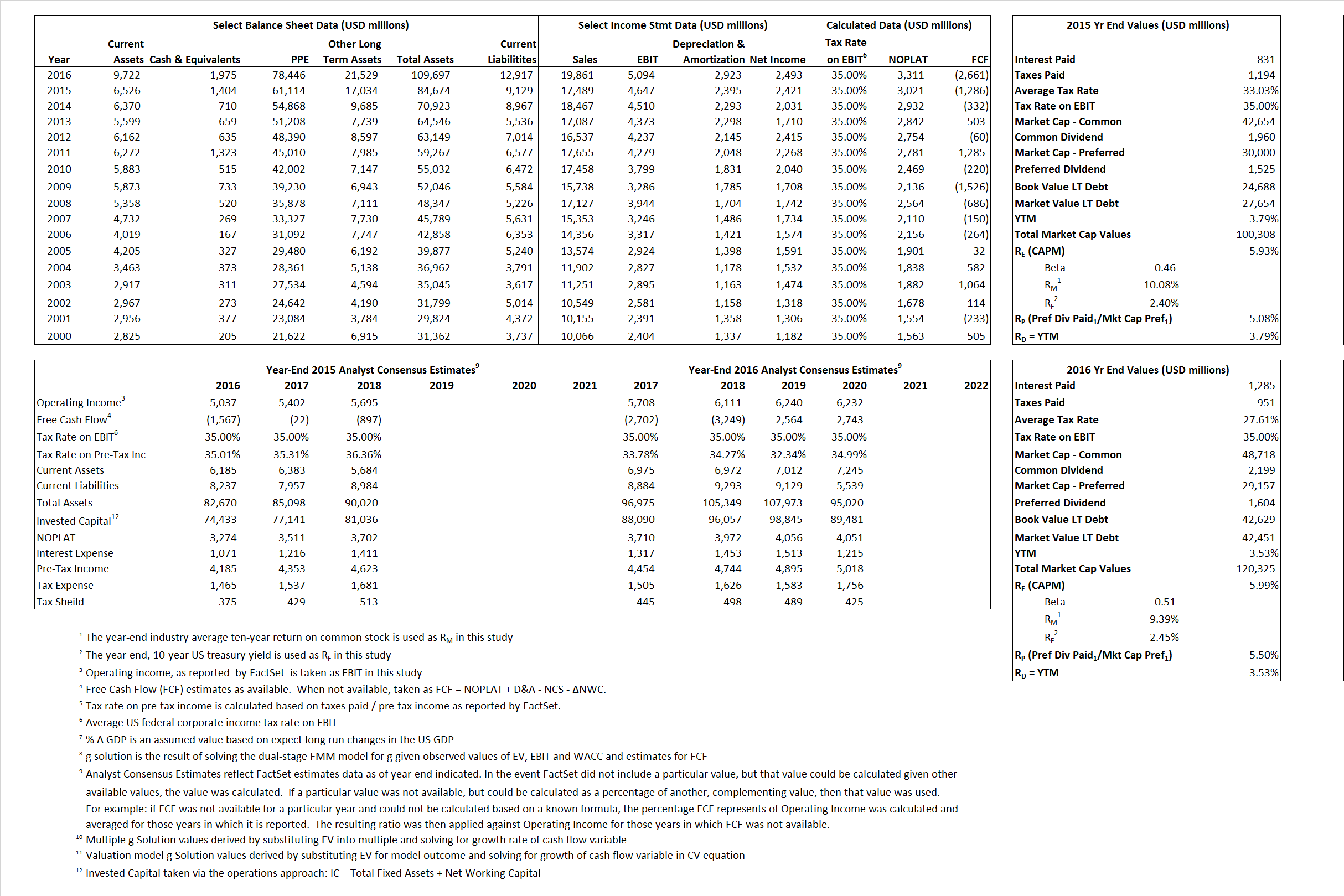# Southern Company

## Analyst Listing

The following analysts provide coverage for the subject firm as of May 2016:

 Broker Analyst Analyst Email SunTrust Robinson Humphrey Ali Agha ali.agha@suntrust.com Jefferies Anthony C. Crowdell acrowdell@jefferies.com Mizuho Securities USA James Von Riesemann james.vonriesemann@us.mizuho-sc.com Wells Fargo Securities Neil Kalton neil.kalton@wellsfargo.com Guggenheim Securities Shahriar Pourreza shahriar.pourreza@guggenheimpartners.com SunTrust Robinson Humphrey Ali Agha ali.agha@suntrust.com

## Primary Input Data## Derived Input Data

### Equational Form

Net Operating Profit Less Adjusted Taxes NOPLAT 3,021 3,311$NOPLAT\, =\, EBIT\, x\, (1 \,-\, Avg \,\,Tax\,\, Rate\,\, on\,\, EBIT)$
Free Cash Flow FCF (1,286) (2,661)$FCF\,=NOPLAT\,+\,Non-Cash\,Expenses-\Delta NWC\,-\,NCS$
Tax Shield TS 274 355$TS\,=\,Interest\,\,Paid\,\,x\,\, Avg \,\,Tax\,\,Rate\,\, on\,\, Pre-Tax\,\, Income$
Invested Capital IC 75,545 96,780$IC\,=\,Fixed\,\,Operating\,\,Assets\,\,+\,\,Net\,\, Working\,\, Capital$
Return on Invested Capital ROIC 4.00% 3.42%$ROIC\,=\,\frac { NOPLAT }{ IC }$
Net Investment NetInv 15,984 24,158$NetInv\,=\,{ {IC}_{1}}-{{IC}_{0}}+Depreciation$
Investment Rate IR 529.18%  729.61%$IR\,=\,\frac {NetInv}{NOPLAT}$
Weighted Average Cost of Capital
WACCMarket 4.74% 4.66%$WACC\,=\,\frac { E }{ V } { R }_{ E }\,+\,\frac { P }{ V } { R }_{ P }\,+\,\frac { D }{ V } { R }_{ D }\left( 1- Avg\,\, Tax\,\,Rate\,\,on\,\,Pre-Tax\,\,Income \right)$
WACCBook   4.09%   4.04%
Enterprise value
EVMarket 98,904 118,350$EV\,=\,Market\,\,Cap\,\,Equity\,+\,\,Long\,\,Term\,\,Debt\,-\,Cash$
EVBook    95,367  118,528
Long-Run Growth
g = IR x ROIC
21.16%  24.96% Long-run growth rates of the income variable are used in the Continuing Value portion of the valuation models.
g = %$\Delta$ GDP   2.50%   2.50%
Margin from Operations M   26.57%   25.65%$M\,\,=\,\,\frac{EBIT}{SALES}$
Depreciation/Amortization Rate D  34.01%  36.46%$D\,\,=\,\,\frac{D+A}{EBITDA}$

## Valuation Multiple Outcomes

The outcomes presented in this study are the result of original input data, derived data, and synthesized inputs.

### model g solution

12/31/2015 12/31/2016 12/31/2015 12/31/2016 12/31/2015 12/31/2016

EV/SALES$\frac {EV}{Sales} \,= \,\frac{ROIC\, -\, g}{ROIC\,(WACC\,-\,g)}\,(1\,-\,T)\,(M)$

5.66  5.96  7.15%  10.21%  6.12%  7.31%

EV/EBITDA$\frac {EV}{EBITDA} \,= \,\frac{ROIC\, -\, g}{ROIC\,(WACC\,-\,g)}\,(1\,-\,T)\,(1\,-\,D)$

14.04 14.76 7.15% 10.21% 6.12% 7.31%

EV/NOPLAT$\frac {EV}{NOPLAT} \,= \,\frac{ROIC\, -\, g}{ROIC\,(WACC\,-\,g)}$

32.74 35.74 7.15% 10.21% 6.12% 7.31%

EV/FCFOPS$\frac {EV}{FCF_{OPS}} \,= \,\frac{ROIC\, -\, g}{ROIC\,(WACC\,-\,g)}\,(1\,-\,T)$

-76.91 -44.48 7.15% 10.21% 6.12% 7.31%

EV/EBIT$\frac {EV}{EBIT} \,= \,\frac{ROIC\, -\, g}{ROIC\,(WACC\,-\,g)}\,(1\,-\,T)$

21.28 23.23 7.15% 10.21% 6.12% 7.31%

EV/IC$\frac {EV}{IC} \,= \,\frac{ROIC\, -\, g}{WACC\,-\,g}$

1.31 1.22 7.15% 10.21% 6.12% 7.31%